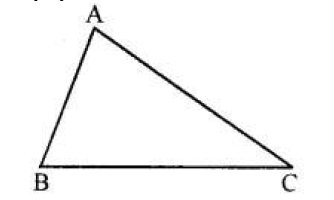# ML Aggarwal Solutions Class 6 Mathematics Solutions for Understanding Symmetrical Shapes Exercise 11 in Chapter 11 - Understanding Symmetrical Shapes

Question 6 Understanding Symmetrical Shapes Exercise 11

In the given figure, measure the lengths of the sides of the triangle ABC and verify:

(i) AB + BC > AC

(ii) BC + AC > AB

(iii) AC + AB > BCFrom the figure,

By using the divider and ruler we measured the given figure,

So,

AB = 2.6 cm, BC = 3.9 cm, AC = 4 cm

(i) AB + BC > AC

2.6 + 3.9 cm > 4 cm

6.5 cm > 4 cm

(ii) BC + AC > AB

3.9 + 4 cm > 2.6 cm

7.9 cm > 2.6 cm

(iii) AC + AB > BC

4 cm + 2.6 cm > 3.9 cm

6.6 cm > 3.9 cm

Related Questions

Lido

Courses

Teachers

Book a Demo with us

Syllabus

Maths
CBSE
Maths
ICSE
Science
CBSE

Science
ICSE
English
CBSE
English
ICSE
Coding

Terms & Policies

Selina Question Bank

Maths
Physics
Biology

Allied Question Bank

Chemistry
Connect with us on social media!